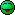# I Why do we need two representations of SU(3)

#### Jelly-bean

Summary
if we use up, down and staring quarks and their own antiparticle we can create the Eightfold way and understand mesons by the hyper charge and isospin projections.
Summary: if we use up, down and staring quarks and their own antiparticle we can create the Eightfold way and understand mesons by the hyper charge and isospin projections.

I don't understand how the conjugate representation of SU(3) allows us to create a vector space of dimension 3, while SU(3) by itself cannot.

Related Quantum Physics News on Phys.org

Staff Emeritus
You have three quarks, each in a triplet representation. 3 x 3 x 3 = 3 x (6 x 3bar) = (3 x 6) + (3 x 3bar) = 10 + 8 + 8 + 1. One of the octets is the eightfold way.

#### strangerep

staring quarks
Now there's a caption begging for a cartoon.•Jelly-bean

#### Jelly-bean

You have three quarks, each in a triplet representation. 3 x 3 x 3 = 3 x (6 x 3bar) = (3 x 6) + (3 x 3bar) = 10 + 8 + 8 + 1. One of the octets is the eightfold way.
sorry I don't think I get the second step. Where did the 6 come from?

Staff Emeritus
Where did the 6 come from?
The algebra of SU(3). 3x3 has 9 elements, and they fall into the sextet and 3bar representations.

#### samalkhaiat

$q^{a} \left( = u , d , s \right) \in \{ 3 \}$, a 3-vector in the fundamental (or defining) representation space $\{ 3 \}$ of $\mbox{SU}(3)$. The 9-component tensor $q^{a}q^{b} \in \{ 3 \} \otimes \{ 3 \}$ can be decomposed as follows $$q^{a}q^{b} = S^{ab} + A^{ab} ,$$ where $$S^{ab} = S^{ba} = \frac{1}{2} \left( q^{a}q^{b} + q^{b}q^{a}\right) ,$$ is symmetric (therefore 6-component) tensor, and $$A^{ab} = - A^{ba} = \frac{1}{2} \left( q^{a}q^{b} - q^{b}q^{a}\right),$$ is anti-symmetric (i.e., 3-component) tensor. Since $S^{ab}$ and $A^{ab}$ don’t mix under $\mbox{SU}(3)$ transformation, they must belong to different representation spaces: $S^{ab} \in D^{6} \equiv \{ 6 \}$, and $A^{ab} \in D^{3} \equiv \{ \bar{3}\}$. In order to complete the proof of $\{ 3 \} \otimes \{ 3 \} = \{ 6 \} \oplus \{ \bar{3} \}$, you need to know the answers to the following 2 questions: The tensor $A^{ab}$ has only 3 independent components, i.e., it belongs to a 3-dimensional vector space, which we denoted by $D^{3}$. So, 1) why did we identify $D^{3}$ with the conjugate representation space $\{ \bar{3} \}$ and not with the space $\{ 3 \}$? In other words, where did the bar on the 3 come from? And 2) how do we know that $D^{6} \equiv \{ 6 \}$ is an irreducible space?

Last edited:

#### vanhees71

Gold Member
From a physics point of view we need it, e.g., for QCD's color-charge space, i.e., we want to have antiquarks carrying the "anticolors" of the quarks' colors.

#### DarMM

Gold Member
Try reading the Appendix on group theory in A. Zee's "Quantum Field Theory in a Nutshell" @Jelly-bean . I know a good few people who found it helpful for clearing up things like why $3\otimes 3 = 6 \oplus \bar{3}$. It's basically about two things:
1. Symmetric and Antisymmetric tensors
2. The Levi-Cevita symbol converting between upper and lower indicies

•vanhees71